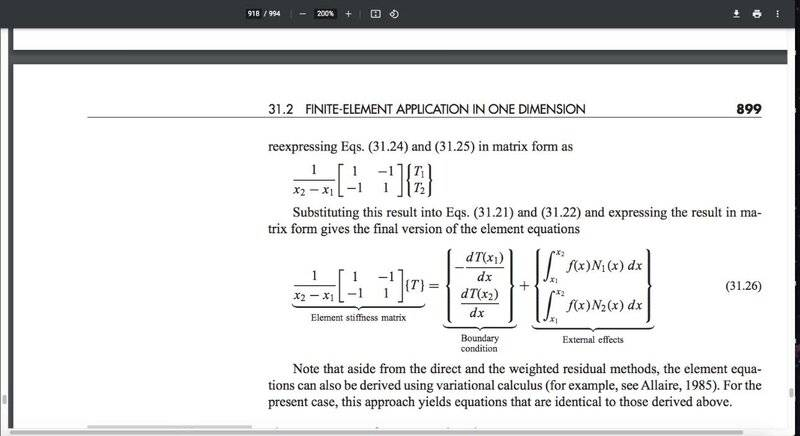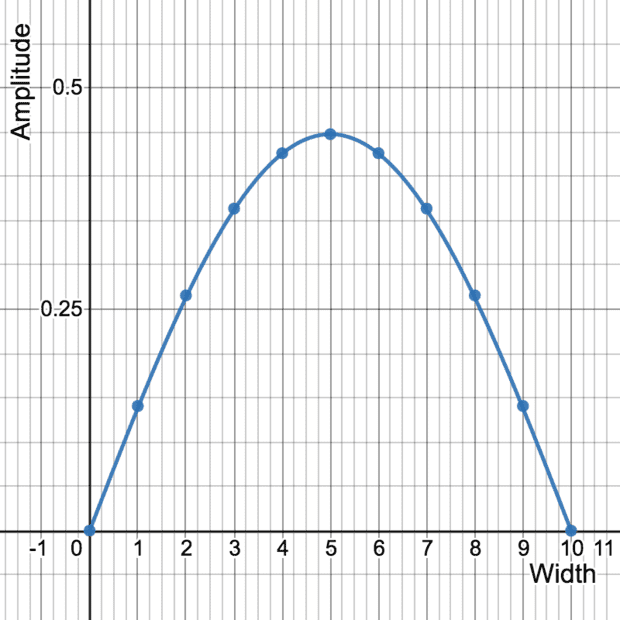# FEM Method for the Wave Equation

• I
• bob012345
In summary, the author is trying to understand how to apply the finite element method for a simple 1D wave equation with four elements. The author assumes that the user knows what the function ##f(x)## is, but if ##f(x)## is ##T(x)## then the equation becomes \begin{multline*}\frac{d^2T}{dx^2} + kT(x) = -f(x).\end{multline*} which can be solved by using the method shown in Numerical Methods for Engineers by Chapra and Canale.f

#### bob012345

Gold Member
TL;DR Summary
How to set up the Finite Element Method of a 1D Wave Equation.
I am trying to understand how to apply the finite element method for a simple 1D wave equation with four elements for learning purposes.

$$\frac{d^2 T(x)}{dx^2} = -f(x)$$

I am stuck because the structure of the equations set up in Numerical Methods for Engineers by Chapra and Canale as shown here seems to assume one knows what the function ##f(x)## is. What if ##f(x)## is ##T(x)## as in the wave equation? Is that case doable? Thanks.•Delta2
I assume $N_i$ are test functions with $T$ approximated by $T_1N_1(x) + T_2N_2(x)$. If so, then $$\frac{d^2T}{dx^2} + kT(x) = -f(x)$$ where $f$ is a known source term gives $$\begin{multline*} \int_{x_1}^{x_2} N_i(x)\frac{d^2T}{dx^2} + kN_i(x)T(x) + N_i(x)f(x)\,dx \\= \left[ N_i(x)\frac{dT}{dx}\right]_{x_1}^{x_2} +\int_{x_1}^{x_2} -\frac{dN_i}{dx}\frac{dT}{dx} + kN_i(x)T(x) + N_i(x)f(x)\,dx \end{multline*}$$ which results in $$\begin{multline*} \begin{pmatrix} \int_{x_1}^{x_2} \left(\frac{dN_1}{dx}\right)^2\,dx & \int_{x_1}^{x_2} \frac{dN_1}{dx}\frac{dN_2}{dx} \,dx \\ \int_{x_1}^{x_2} \frac{dN_1}{dx}\frac{dN_2}{dx} \,dx & \int_{x_1}^{x_2} \left(\frac{dN_2}{dx}\right)^2\,dx \end{pmatrix} \begin{pmatrix} T_1 \\ T_2 \end{pmatrix} \\ - k\begin{pmatrix} \int_{x_1}^{x_2} N_1^2(x)\,dx & \int_{x_1}^{x_2} N_1(x)N_2(x)\,dx \\ \int_{x_1}^{x_2} N_1(x)N_2(x)\,dx & \int_{x_1}^{x_2} N_2^2(x)\,dx \end{pmatrix} \begin{pmatrix} T_1 \\ T_2 \end{pmatrix} \\ = \begin{pmatrix} N_1(x_2) \frac{dT}{dx}(x_2) - N_1(x_1) \frac{dT}{dx}(x_1) \\ N_2(x_2) \frac{dT}{dx}(x_2) - N_2(x_1) \frac{dT}{dx}(x_1) \end{pmatrix} + \begin{pmatrix} \int_{x_1}^{x_2} N_1(x)f(x)\,dx \\ \int_{x_1}^{x_2} N_2(x)f(x)\,dx \end{pmatrix}.\end{multline*}$$ I'm sure the authors will give an example of this at some point.

The wave equation is actually a PDE, so $T_1$ and $T_2$ are not constants but functions of time. You therefore end up with the system of ODEs $$A\begin{pmatrix} \frac{d^2T_1}{dt^2} \\ \frac{d^2T_2}{dt^2} \end{pmatrix} = -c^2 B \begin{pmatrix} T_1 \\ T_2 \end{pmatrix} + (\mbox{boundary terms})$$ where $B$ is the stiffness matrix and $$A = \begin{pmatrix} \int_{x_1}^{x_2} N_1^2(x)\,dx & \int_{x_1}^{x_2} N_1(x)N_2(x)\,dx \\ \int_{x_1}^{x_2} N_1(x)N_2(x)\,dx & \int_{x_1}^{x_2} N_2^2(x)\,dx \end{pmatrix}.$$

•Delta2 and bob012345
I worked it out and it works great! Thanks. Here is a plot of the points calculated by the Finite Element Method as compared to the exact analytical solution ##\psi(x)= \sqrt{\frac{2}{10}}sin(\frac{\pi x}{10})## of an infinite potential well of width 10 units.;Last edited:
•Delta2
What does the input function ##f(x)## represent in the case of a wave equation? I know from experience it is a constant and it is necessary to solve the linear equations. I also noticed it can be scaled to get the amplitude I want but I am having trouble justifying it and what value to give it.